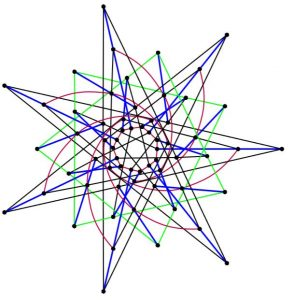By James Evans, University of Western Australia

A generalised n-gon is a finite configuration of points and lines obeying the following conditions:

• for all k<n there are no ordinary k-gons in the geometry
• there are lots of ordinary n-gons in the geometry
• the system is ‘non-degenerate’

Collectively the generalised n-gons are called generalised polygons.An example of a finite generalised 6-gon/hexagon

They may seem basic, but a lot emerges from these simple axioms. For example, each polygon has a pair of numbers, (s,t) such that every line contains s+1 points and every point touches t+1 lines. There are severe restrictions on the values of s and t. An important result is the Feit-Higman theorem: generalised n-gons only exist for n = 2, 3, 4, 6 and 8. These sorts of results show that the polygons have a highly regular but also tightly constrained structure, suggesting that they are rare and exotic objects.

The most compelling and surprising aspect of the polygons is their symmetry. The primary examples, known as the classical polygons, are absurdly symmetric. Their symmetry groups are huge compared to their sizes and obey very strong conditions. But most curiously, their symmetry groups are among the infamous finite simple groups.

There are many mysteries surrounding the polygons and their symmetries. My research focused on this one: have we found all generalised polygons whose symmetry groups are simple groups?

The main method of progress is the following. Start with all groups obeying some condition. Then using the powers of group theory, number theory and more, try to determine which ones are the symmetries of some polygon (without knowing the polygons they are the symmetries of!). In many cases it has been shown that if a polygon obeys certain (even quite weak) conditions, then its symmetry group must be (almost) simple. This is a baffling and exceptional result: why would even weak conditions force the symmetry group to be simple? It is not at all clear what about the axioms, which seemingly have nothing to do with symmetry, could force this. Answering that question is the eventual end goal of this whole effort.

Once the restrictions on the symmetry groups have been found, the next step is to use these to learn about the polygons themselves: to find examples, to derive general facts about their structure, etc. This placement made a small contribution here. We created a program which takes in any finite group and produces all 4-gons which it ‘acts on point-primitively’ (whatever that means). This was used to rule out a troublesome case which had avoided theoretical treatment.

Despite all of the progress that has been made so far, the generalised polygons remain remarkable and puzzling objects. Indeed, it seems that most of what we have learned boils down to ‘the polygons are more remarkable and puzzling than we thought’. But this is probably for the best: if mathematicians were able to completely understand the polygons, then they would no longer have such an interesting and difficult problem to investigate.

James Evans was a recipient of a 2018/19 AMSI Vacation Research Scholarship.